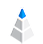# Correlation Matrix and Table

## What is a Correlation Matrix?

We already know that a correlation shows the mutual relationship between two or more factors. Do not forget that correlation is not equal to causation. However, what is a correlation matrix? A correlation matrix is a table that shows the correlation coefficients between variables. In the table, you will find the values of correlation and see which pairs have the highest correlation.

Tip: The value in the diagonal of the table is always 1, because the correlation between a variable and itself is 1.

## What Does a Correlation Matrix Look Like?

In our application, you will find the correlation matrix under Relation. Every column is a variable in the table, and the correlations are shown between them. It is like a relation map, but here you will see the strength of the relations as numbers. The higher the number, the stronger the correlation between the variables.Tip: The darker the field, the stronger the relationship between the variables.

If you click on the icon at the top of a column, then that column will be ordered by strength. Doing so will help you quickly find the strongest relationship in each column. Just like our other features, the correlation matrix is more than a simple table. You can click on any value in the table, and the correlation will be visualized on charts.

## Search and Filter

We know that you will sometimes have more than 100 columns in your spreadsheet, and you do not want to see all of them in your correlation matrix. The good news is that you do not have to. On the left sidebar, you can pick only the columns that you would like to see in the correlation matrix. You can also search between your columns by typing the column’s name into the search field.Can we elevate this? Of course, we can! Filtering is also available here. You can set one or more filters to analyze a preferred argument. In the matrix, only the filtered data will be shown. If you want to see the unfiltered data, just hit Pause in the left sidebar.

Any changes that you make here - selecting columns and filtering - will be used if you switch between menus (Charts, Relation Map, and so on).

## Relation Table

A relation table is like a correlation matrix. It shows the correlation coefficients between your columns. However, there are some differences between the two visualization. With a relation table, the relations are listed, and a short explanation is next to each number. The colors have also meanings here. Blue means that, there is a positive correlation, and orange means that there is a negative correlation. Just like in the matrix, the darker the color, the stronger the relation.## Summary

If you are a man of numbers and you trust more in them, then this will be the most useful feature for you. The table shows you the strength of correlation in numbers, and you can visualize it on charts or in the relation map at any time.